# 不需再用数据**表，这个万能的Excel函数更好用！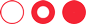Sumproudct函数1、本年累计公式

=SUMPRODUCT((YEAR(A2:A182)=H1)*E2:E182)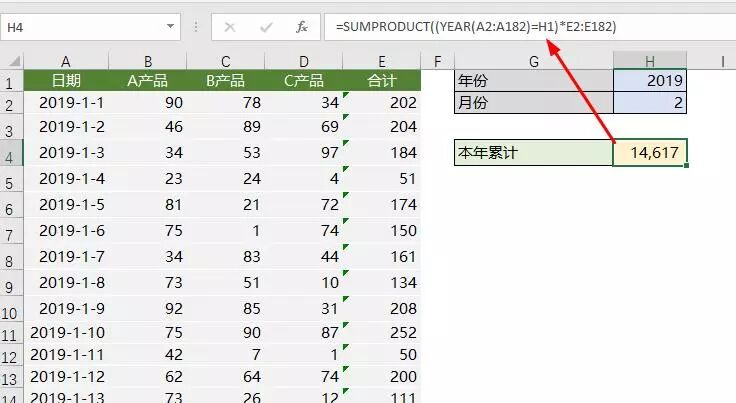2、本月累计公式

=SUMPRODUCT((YEAR(A2:A182)=H1)*(MONTH(A2:A182)=H2)*E2:E182)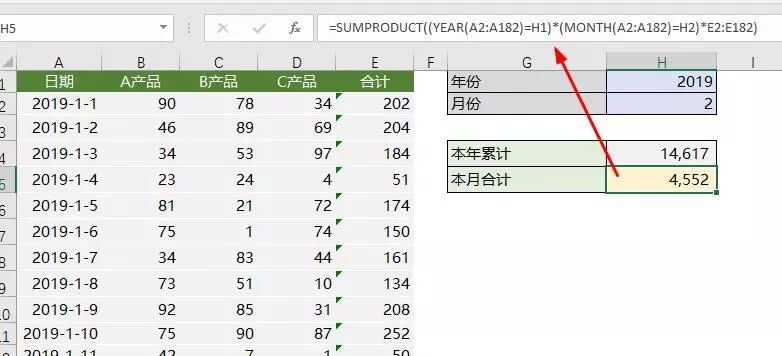3、本月已统计天数本月平均公式

=SUMPRODUCT((YEAR(A2:A182)=H1)*(MONTH(A2:A182)=H2)*(B2:B182""))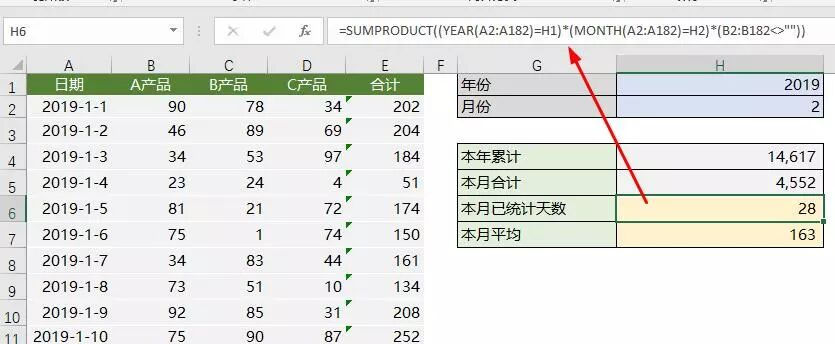4、最近一天公式

=LOOKUP(1,0/(B2:B173""),A2:A173)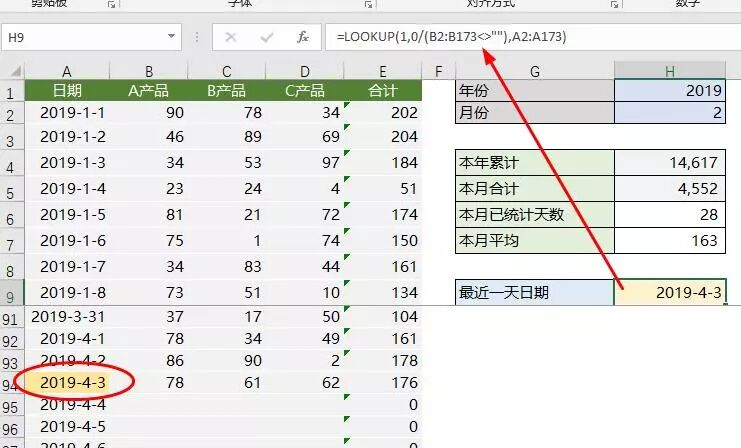5、最近30天销售合计平均公式

=SUMPRODUCT(((A2:A182)>=H9-30)*E2:E182)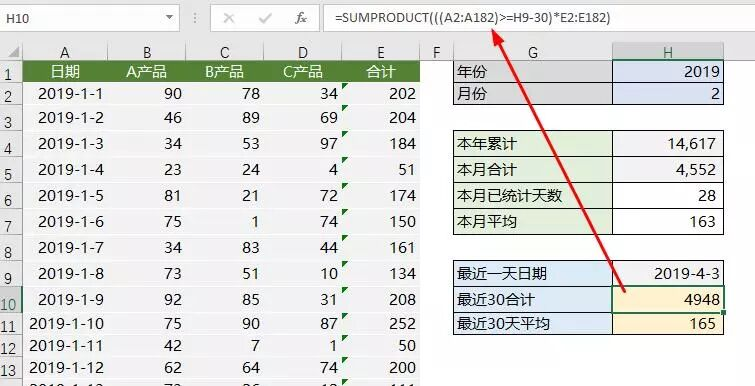6、本日（最后一天）销售金额公式

=LOOKUP(1,0/(D2:D173""),E2:E173)7、本日销售排名（本年）公式

=SUMPRODUCT((YEAR(A2:A182)=YEAR(H9))*(E2:E182>H12))+1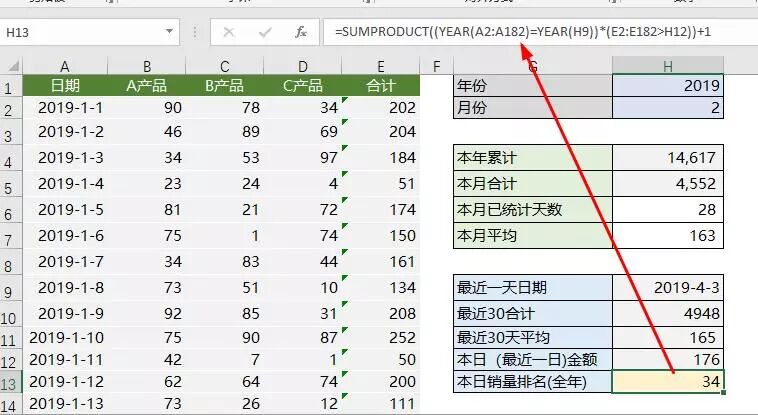8、本日销售排名（本月）公式

=SUMPRODUCT((YEAR(A2:A182)=YEAR(H9))*(MONTH(A2:A182)=MONTH(H9))*(E2:E182>H12))+1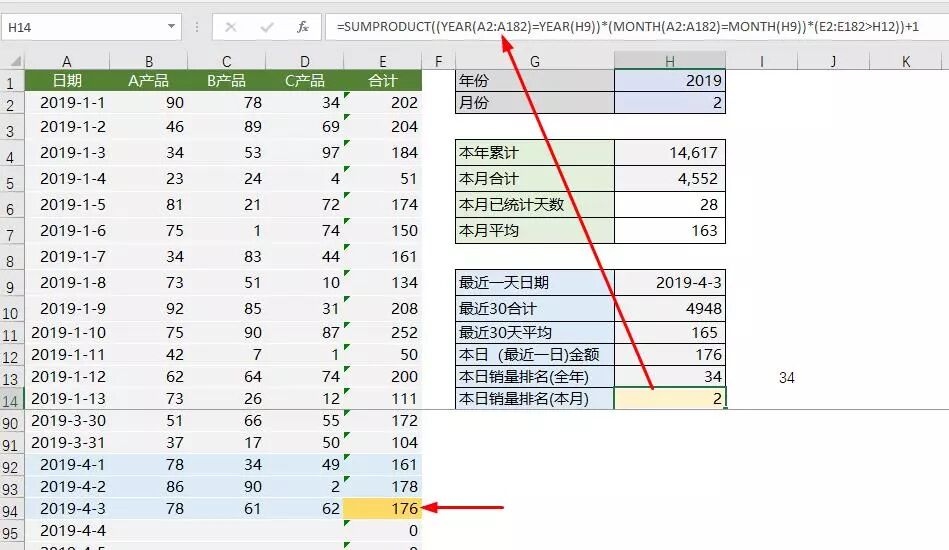【免责声明】：本公众号对转载、分享的内容、陈述、观点判断保持中立,不对所包含内容的准确性、可靠性或完善性提供任何明示或暗示的保证,仅供读者参考！

4月1日起，这12种费用发票不能再报销了！一旦收到要立即退回，否则损失要由会计承担！▼更多精彩内容，请关注我们▼把时间交给阅读